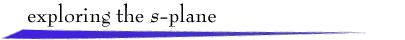For a continuous-time, linear, time-invariant system with transfer function Y(s)/R(s) = G(s) and a unit-step input signal, R(s) = 1/s, the Laplace transform of the response is Y(s) = G(s)/s. If the system is stable, the steady-state value of the response will be y(t) = G(0). This is called the dc-gain of the system. To view the unit-step response y(t) for the transfer function

K (s-z1)(s-z2)...(s-zm)
G(s) = ---------------------
(s-p1)(s-p2)...(s-pn)
click the Pole button and then click on the s-plane to locate a pole, or complex pair of poles. This can be repeated to add a total of no more than six poles. Zeros are added in a similar fashion, with the condition that there be more poles than zeros. The default gain K preserves G(0) = 1, but the user can enter a desired gain value.

To see the unit-step response change as a pole or zero is moved, click Move and drag the pole or zero. (Remember that the default gain value changes to maintain G(0) = 1.)

 You need a Java-compatible browser to see the demo.return to demonstrations page

 Applet by Brian Woo.